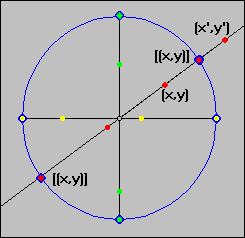#Interactive Real Analysis

Next | Previous | Glossary | Map

## 1.3. Equivalence Relations and Classes

### Example 1.3.5:

Consider the set R x R \ {(0,0)} of all points in the plane minus the origin. Define a relation between two points (x, y) and (x', y') by saying that they are related if they are lying on the same straight line passing through the origin.

This relation is an equivalence relation, and the resulting space of equivalence classes is called projective space. By picking an appropriate member for each class one can find a graphical representation for this space.

As usual, we need to check to first verify that this is a relation, and then check the three conditions that turn a relation into an equivalence relation: This clearly is a relation (most things are) so that we will have to check:
reflexive:
every point is related to itself, since it lies on a line through the origin.
symmetry:
if (x, y) ~ (x', y'), then they are both on the same line through the origin. This is a symmetric definition, so symmetry holds as well.
transitivity:
here we should use some math. Recall that two points (x, y) and (x', y') are on the same line through the origin, if and only if there is a non-zero real number t such that (x, y) = t (x', y').
• take (x, y) ~ (x', y').
Then there exists t # 0 with (x, y) = t (x', y').
• take (x', y') ~ (x'', y'')
Then there exists s # 0 with (x', y') = s (x'', y''). But then (x'', y'') = 1/s (x', y') = 1 / (s t) (x, y), hence (x'', y'') ~ (x, y), proving transitivity.
This proves that the relation is indeed an equivalence relation. Next, we have to try to find a graphical representation of P by picking an appropriate member for each class.

Since the relation is an equivalence relation we know that there are disjoint equivalence classes [(x, y)], and we can pick any member of [(x, y)] as a representative of that class. Define a point p on the unit circle to be equal to the whole equivalence class [(x, y)] containing p. That is, we identify each equivalence class with a point on the unit circle by drawing the line through that point and the origin. That line contains every member of the equivalence class.

Numbers on the unit circle that are diagonally opposite each other, however, are in the same equivalence class, since they are on the same line through the origin.Thus we identify the space P with the unit circle, where diagonally opposite numbers are identical. Here is a poor picture of this space:This space is called projective space, and can be generalized to higher dimensions as well.
Next | Previous | Glossary | Map